Question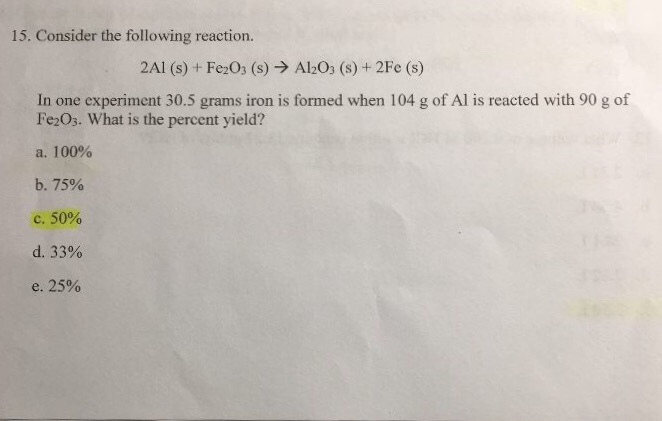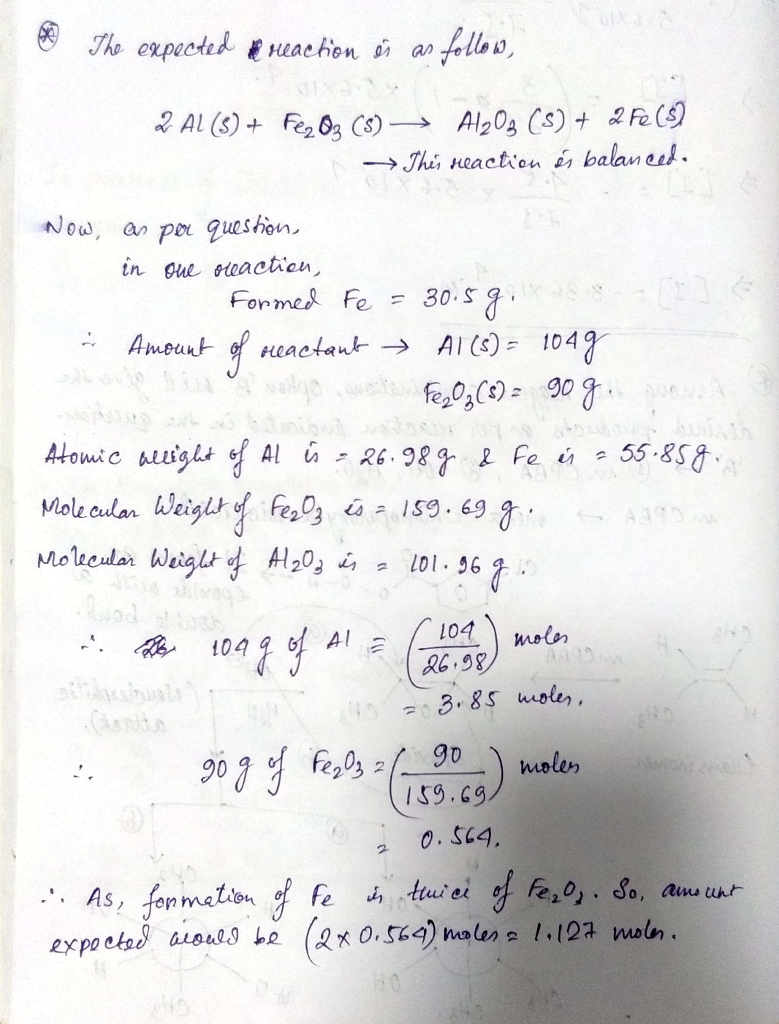2nd page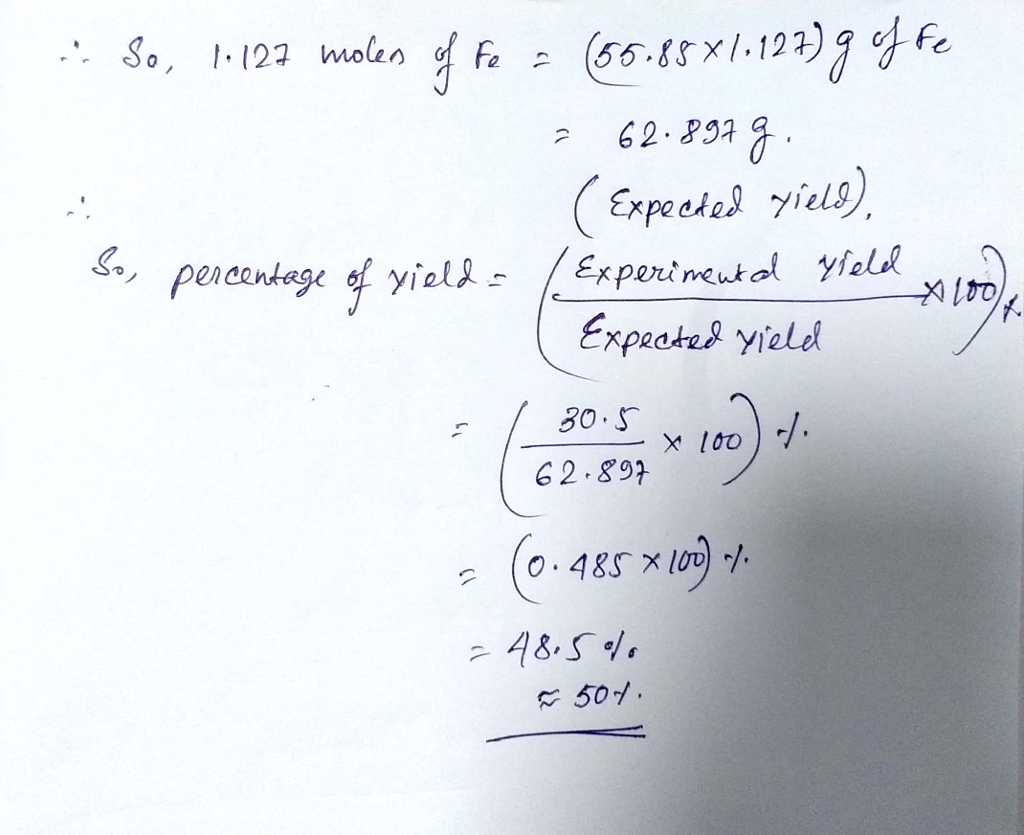#### Earn Coins

Coins can be redeemed for fabulous gifts.

Similar Homework Help Questions
• ### Consider the following balanced chemical reaction Fe2O3(s) + 2Al(s) → 2Fe(s) + Al2O3(s) What mass of...

Consider the following balanced chemical reaction Fe2O3(s) + 2Al(s) → 2Fe(s) + Al2O3(s) What mass of iron can be produced if 50.0 g of Fe2O3 is mixed with 40.0 grams of Al?

• ### 10.Calculate the standard enthalpy change for the reaction 2Al(s) + Fe2O3(s) →2Fe(s) + Al2O3(s) given that...

Calculate the standard enthalpy change for the reaction 2Al(s)+Fe2O3(s) → 2Fe(s)+Al2O3(s)given that2 Al(s)+ 3/2O2(g) → Al2O3(s)  ΔHrxn°=-1669.8 kJ/mol2 Fe(s)+3/2O2(g) → Fe2O3(s) ΔHrxn°=-822.2 kJ / mol

• ### 4 Consider the balanced chemical equation: 2Al(s) + Fe2O3(s) — 2Fe(s) + Al2O3(s) heat of reaction...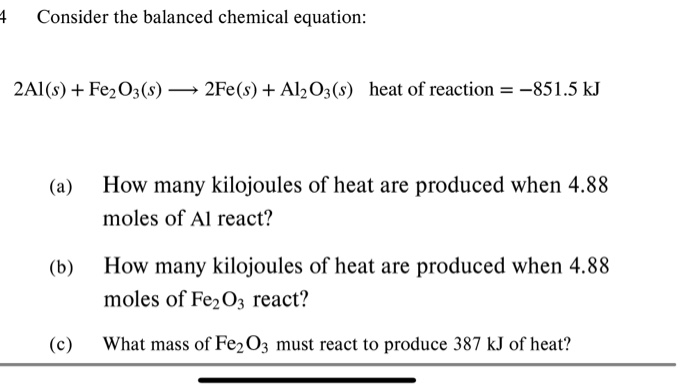4 Consider the balanced chemical equation: 2Al(s) + Fe2O3(s) — 2Fe(s) + Al2O3(s) heat of reaction = -851.5 kJ (a) How many kilojoules of heat are produced when 4.88 moles of Al react? (b) How many kilojoules of heat are produced when 4.88 moles of Fe2O3 react? (c) What mass of Fe2O3 must react to produce 387 kJ of heat?

• ### consider the following reaction 2Fe + Al2o3-> 2al fe2o3 what mass is produced when 5g of...

consider the following reaction 2Fe + Al2o3-> 2al fe2o3 what mass is produced when 5g of Fe and 5g of al2o3 react

• ### Consider the reaction: Fe2O3(s) + 2Al(s)Al2O3(s) + 2Fe(s) Using standard absolute entropies at 298K, calculate the...

Consider the reaction: Fe2O3(s) + 2Al(s)Al2O3(s) + 2Fe(s) Using standard absolute entropies at 298K, calculate the entropy change for the system when 2.37 moles of Fe2O3(s) react at standard conditions. S°system = ____J/K

• ### Given that: 2Al(s)+(3/2)O2(g)--->Al2O3(s) change in H(rxn)= -1601 kJ/mol 2Fe(s)+(3/2)O2(g)--->Fe2O3(s) change in H(rxn)= -821 kJ/mol Calculate the standard enthalpy change for the following reaction: 2Al(s)+Fe2O3(s)--->2Fe(s)+Al2O3(s)

Given that: 2Al(s)+(3/2)O2(g)--->Al2O3(s) change in H(rxn)= -1601 kJ/mol 2Fe(s)+(3/2)O2(g)--->Fe2O3(s) change in H(rxn)= -821 kJ/mol Calculate the standard enthalpy change for the following reaction: 2Al(s)+Fe2O3(s)--->2Fe(s)+Al2O3(s) _______kJ

• ### Iron oxide reacts with aluminum in an exothermic reaction. Fe2O3(s) + 2 Al(s) → 2...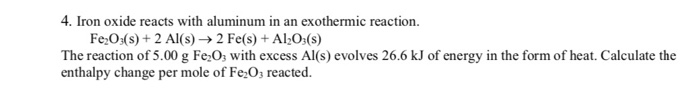Iron oxide reacts with aluminum in an exothermic reaction. Fe2O3(s) + 2Al(s) → 2Fe(s) + Al2O3(s) The reaction of 5.00g Fe2O3 with excess Al(s) evolves 26.6 kJ of energy in the form of heat. Calculate the enthalpy change per mole of Fe2O3 reacted.

• ### Under what conditions will the reaction be spontaneous? (a) Al2O3(s)+2Fe(s)→Fe2O3(s)+2Al(s)ΔS>0 and ΔH>0 (b) CS 2 (...

Under what conditions will the reaction be spontaneous? (a) Al2O3(s)+2Fe(s)→Fe2O3(s)+2Al(s)ΔS>0 and ΔH>0 (b) CS 2 ( g ) → CS 2 ( l ) Δ S < 0 and Δ H < 0

• ### help !! QUESTION 8 Consider the reaction: Fe2O3 + 2AI 2Fe + Al2O3 In this reaction,...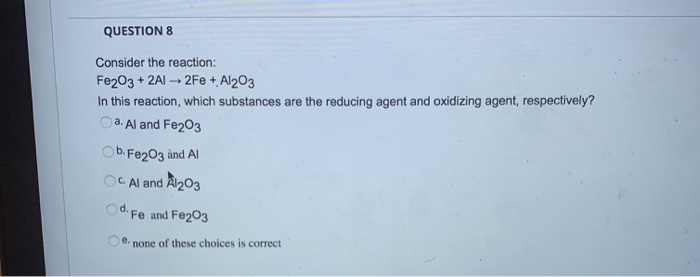help !! QUESTION 8 Consider the reaction: Fe2O3 + 2AI 2Fe + Al2O3 In this reaction, which substances are the reducing agent and oxidizing agent, respectively? a. Al and Fe2O3 b. Fe2O3 and Al OC. Al and A1203 Od. Fe and Fe203 e none of these choices is correct

• ### Consider the reaction Fe2O3(s) + 2Al(s)Al2O3(s) + 2Fe(s) for which H° = -851.5 kJ and S° = -37.00 J/K at 298.15 K. (1) C...

Consider the reaction Fe2O3(s) + 2Al(s)Al2O3(s) + 2Fe(s) for which H° = -851.5 kJ and S° = -37.00 J/K at 298.15 K. (1) Calculate the entropy change of the UNIVERSE when 2.108 moles of Fe2O3(s) react under standard conditions at 298.15 K. Suniverse = J/K (2) Is this reaction reactant or product favored under standard conditions? (3) If the reaction is product favored, is it enthalpy favored, entropy favored, or favored by both enthalpy and entropy? If the reaction is...